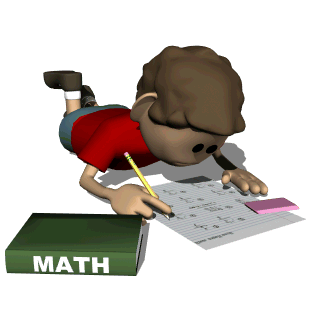•GVSD has provided Engage NY Support to help families and students with our fraction unit.

Students will receive information explaining how to access the math textbook online soon after the year begins.
NEW - To access all math materials online, follow these steps:
1. Go to https://www-k6.thinkcentral.com
2. Enter information for State, District, School.  Check the "Remember my information" box
password - Kdm260000 (capital K, lowercase dm, lunch account number)
4. Click on My Library for access to the text, workbook, and virtual math manipulatives, lesson videos, and interactive games!

Topics of study this year will include:
• whole number and decimal place value
• addition and subtraction of decimals and whole numbers
• multiplication and division of whole numbers and decimals
• measurement in metric and standard units
• fraction and mixed-number operations
• solving algebraic equations following Order of Operations and using operational properties
• geometry
• graphs
• problem-solving.
Links for practicing important math concepts!

Practice using a protractor here

Practice factors and multiples here